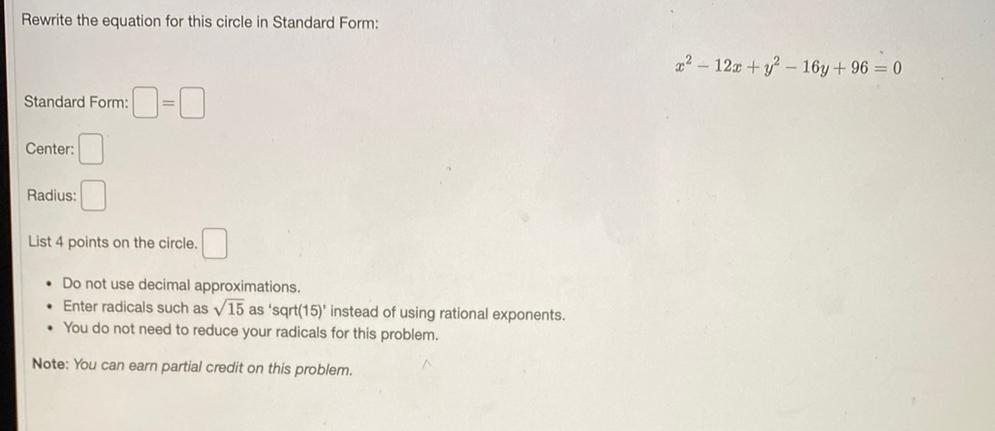Question:

# Rewrite the equation for this circle in Standard Form: 32 - 12x + y2 - 16y + 96 = 0 Standard Form: O=0 Center: Radius: List 4 poRewrite the equation for this circle in Standard Form: 32 - 12x + y2 - 16y + 96 = 0 Standard Form: O=0 Center: Radius: List 4 points on the circle. . Do not use decimal approximations, • Enter radicals such as 15 as 'sqrt(15) instead of using rational exponents. • You do not need to reduce your radicals for this problem. Note: You can earn partial credit on this problem.